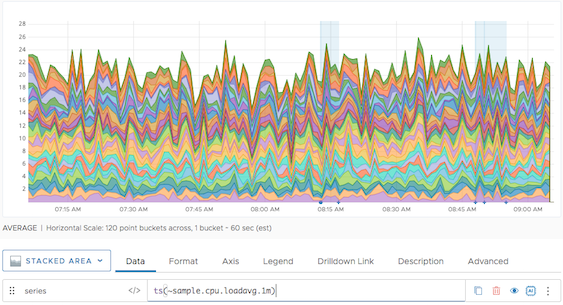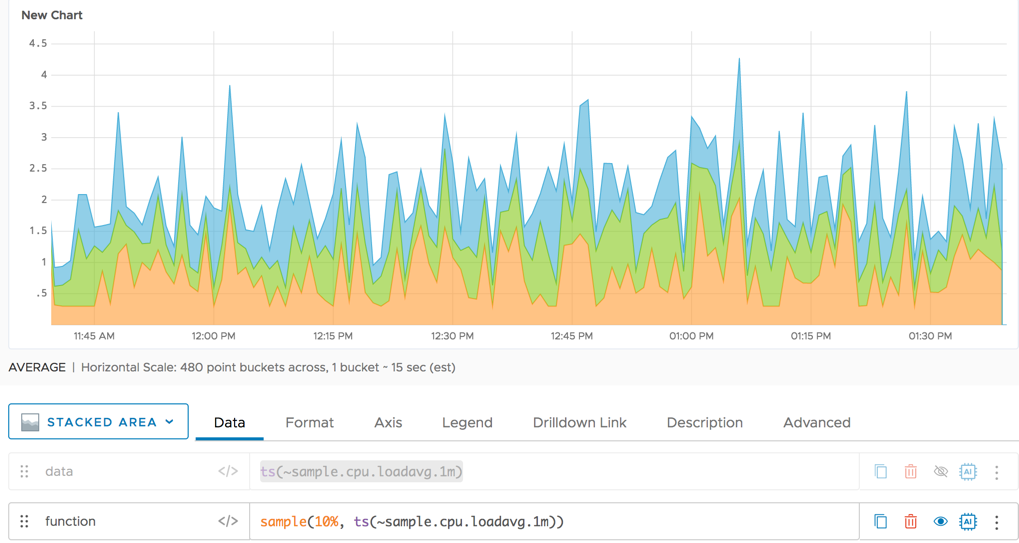Reference to the sample() function

## Summary

``````sample(<numberOfTimeSeries>, <tsExpression>)
``````

Returns `numberOfTimeSeries` non-random time series based on the expression. This function is deterministic as long as the underlying set of time series stays the same. However, the returned values can change, for example, if a new source starts reporting the metric.

## Parameters

ParameterDescription
numberOfTimeSeries Number of time series that you want. You can express this parameter as a number (e.g. 10) or a percentage (e.g. 17%).
tsExpression Expression that you want to filter.

## Description

Returns `numberOfTimeSeries` non-random time series based on the expression. This function is deterministic as long as the underlying set of time series stays the same. The returned values might change, for example, if a new source starts reporting the metric.

Don’t confuse `sample()` with `downsample()`, which returns the values in the expression that occur in each time window.

## Examples

The following example shows first the result of a query that returns a fairly large number of time series.We can use `sample()` to return 10% the time series, in this example, 3.